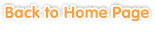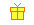Sample questions are shown below. Just click to start!• Chapter 1
Multiplication of Decimals
•
Multiplying Decimals with Whole Numbers•• Chapter 2
Division of Decimals
•
Dividing Decimals with Whole Numbers•• Chapter 3
Repeating Decimals• Chapter 4
Problem Solving: Multiplying and Dividing Decimals• Chapter 5
Factors and Multiples
•
Basis of Factors and Multiples•
Identifying Even and Odd Numbers•
Prime and Composite Numbers•• Chapter 6
Fractions
•
Proper Fractions, Improper Fractions, and Mixed Numbers•
Reducing Fractions•
Finding Common Denominators•
Comparing Fractions•
Adding and Subtracting Fractions with Equal Denominators•
Adding and Subtracting Fractions with Uncommon Denominators•• Chapter 7• Chapter 8
Algebra
•
Evaluating Expressions•
Writing Algebraic Expressions••
Solving One-Step Equations•• Chapter 9
Geometry
•
Solid Shapes•••
Understanding Rectangular Prisms and Cubes••
Converting Units of Area and Volume• Chapter 10
Statistics and Probability
••
Mean, Median, Mode, and Range•
Problem Solving: mean, range, median